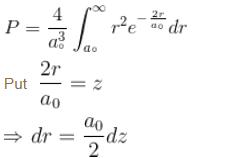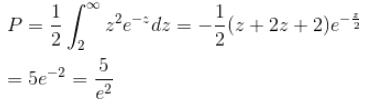Courses

# Quantum Mechanics In Three Dimensions NAT

## 10 Questions MCQ Test Topic wise Tests for IIT JAM Physics | Quantum Mechanics In Three Dimensions NAT

Description
This mock test of Quantum Mechanics In Three Dimensions NAT for IIT JAM helps you for every IIT JAM entrance exam. This contains 10 Multiple Choice Questions for IIT JAM Quantum Mechanics In Three Dimensions NAT (mcq) to study with solutions a complete question bank. The solved questions answers in this Quantum Mechanics In Three Dimensions NAT quiz give you a good mix of easy questions and tough questions. IIT JAM students definitely take this Quantum Mechanics In Three Dimensions NAT exercise for a better result in the exam. You can find other Quantum Mechanics In Three Dimensions NAT extra questions, long questions & short questions for IIT JAM on EduRev as well by searching above.
*Answer can only contain numeric values
QUESTION: 1

### A stone with mass 1kg is whirled in a horizontal circle of radius 1m with a period of revolution equal to 1s. What value of orbital angular momentum quantum no. l describes this motion? (in 1034)

Solution:

The speed of the stone in its orbit is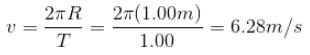Angular momentum = mvr = 1kg × 6.28 m/s × 1m
= 6.28 kg m2/s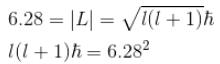For classical objects the quantum numbers are excessively big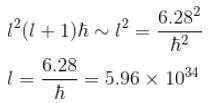*Answer can only contain numeric values
QUESTION: 2

### Calculate the minimum value of for the stone mentioned in problem 4.

Solution: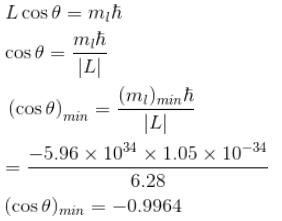*Answer can only contain numeric values
QUESTION: 3

### Calculate the average distance of an electron from the nucleus in the ground state of hydrogen atom is unit of a0.

Solution: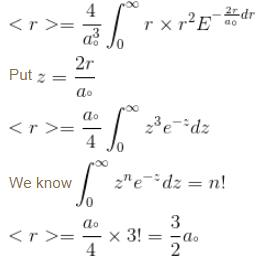*Answer can only contain numeric values
QUESTION: 4

Find the level of degeneracy for particle in a 3d box with energy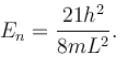Solution: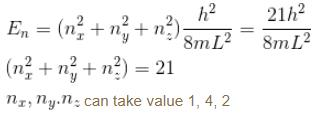level of degeneracy = 6

*Answer can only contain numeric values
QUESTION: 5

Find the value of the normalisation constant in the unit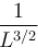of that normalizes the ground state wavefunction of the particle in a box of lengths L, 2L, 3L in  x, y and z directions respectively. Take  √3 = 1.732

Solution:

The probability density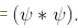Integration over the volume of the cube must be unity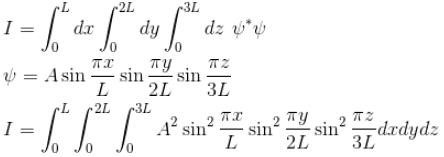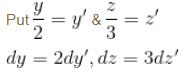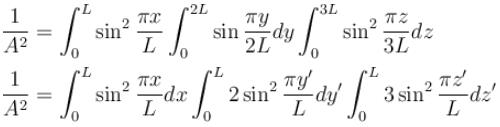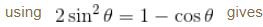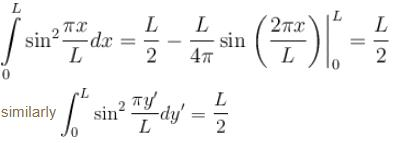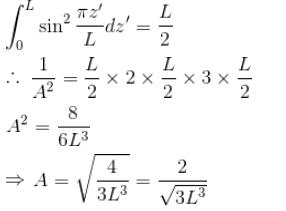*Answer can only contain numeric values
QUESTION: 6

Find the level of degeneracy for a 3 dimensional isotropic quantum harmonic oscillator of energy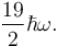Solution: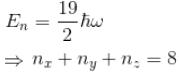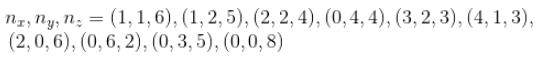(0, 0, 8) 3 states
(2, 2, 4) 3 states
(3, 3, 2) 3 states
(1, 1, 6) 3 states
(4, 4, 0) 3 states
=  3 × 5 = 15 states
Rest will give 6 states each
(0, 1, 7)(1, 2, 5)(4, 1, 3)(2, 0, 6)(0, 3, 5)
6 × 5 = 30
Total = 15 + 30 = 45 states

*Answer can only contain numeric values
QUESTION: 7

Consider two harmonic oscillators in 3 dimensions. For what ratio of angular frequencies will the first excited state of one oscillator have energy twice that of the second oscillator’s third excited state energy i.e find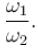Solution: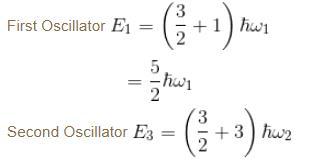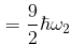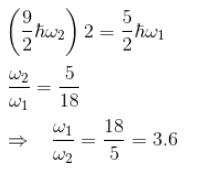*Answer can only contain numeric values
QUESTION: 8

Modeling a defect trap in a crystal as a 3d box with edge length 5Å, find the values of energy for an electron bound to the defect site, assuming the electron to be in the ground state. (in eV)

Solution: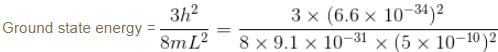= 0.0716 × 10-68 + 31 + 20
= 0.0714 × 10-17 Joules
= 0.0447 × 102 eV
= 4.5 eV

*Answer can only contain numeric values
QUESTION: 9

Find the probability of finding a particle in a 3d box of length L, in the volume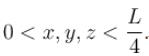(Assume the particle to be in the ground state).

Solution: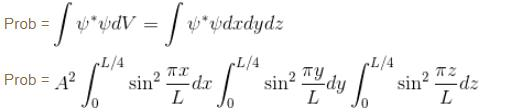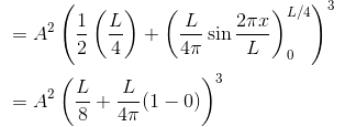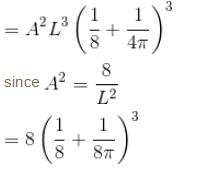= 0.04

*Answer can only contain numeric values
QUESTION: 10

Calculate the probability that the electron in the ground state of hydrogen will be found outside the first Bohr radius in the unit e–2. Take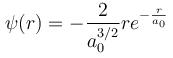Solution:

The probability is found by integrating the radial probability density for this state from  a0  to ∞.